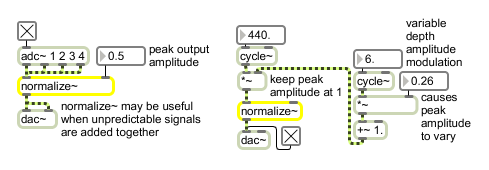# normalize~

Scale on the basis of maximum amplitude

## Description

normalize~ performs real-time normalization of its input by multiplying each input sample value by a scaling factor - computed as the maximum output value (sent either as a signal or a float in the right inlet) over the maximum signal input value received thus far. You can change the maximum input value with the reset message or with a float in the left input. If no argument follows "reset" the new maximum input value (and the initial maximum input value) is 0.000001.

## Arguments

Name Type Opt Description
initial-maximum-output-amplitude float opt The initial maximum output amplitude. The default is 1.

## Messages

 int maximum-output-amplitude [int] Converted to float. float maximum-output-amplitude [float] In right inlet: The maximum output amplitude may be sent as a float instead of a signal. If a signal is connected to the right inlet, a float received in the right inlet is ignored. reset maximum-input-amplitude [float] In left inlet: The word reset, followed by a number, resets the maximum input amplitude to the number. If no number follows reset, or if the number is 0, the maximum input amplitude is set to 0.000001. signal In left inlet: The input signal is normalized -- scaled so that its peak amplitude is equal to a specified maximum. In right inlet: The maximum output amplitude; an over-all scaling of the output.

## Output

signal: The input signal is scaled by the maximum output amplitude divided by the maximum input amplitude.

## ExamplesWhen precise scaling factor varies or is unknown normalize~ sets peak amplitude